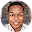# BECE 2020 Mathematics Paper 1OBJECTIVE TEST

Question 1: Simplify ¾ - ⅓ + ⅟₁₂
A. ⅓
B. ½
C. ⅙
D. ⅔

Question 2: Given that N ={x:x is a factor of 18} and M = {x:x is a multiple of 12}, find N⋂M.
A. {1,2,3,6}
B. {1,2,3,6,12}
C. {2,3,6,12,18}
D. {}

Question 3: Express 4382.93 in standard form.
A. 438293 x 10⁴
B. 43.8293 x 10²
C. 4.38293 x 10⁴
D. 4.38293 x 10³

Question 4: Which property of arithmetic is used in a(x+ y) =ax+ ay.
A. Associative
B. Commutative
C. Distributive
D. Initiative

Question 5: Subtract (7x – 3) from (5 – 3x).
A. 10x – 8
B. 4x – 8
C. 8 – 10x
D. 2 – 10x

Question 6: The cost of 12 note books is GH¢ 54.84. find the cost of one note book
A. GH¢ 5.57
B. GH¢ 4.67
C. GH¢ 4.57
D. GH¢ 3.57

Question 7:

Question 8: Simplify: (2ab ²)2 x 3a³b.
A. 6a⁴b⁵
B. 12a³b⁴
C. 12a⁶b⁴
D. 12a⁵b⁵

Question 9: Which of the following polygons does not have a line of symmetry?
A. Kite
B. Isosceles triangle
C. Trapezium
D. Rhombus

Question 10: A trader sold a radio set for GH¢ 72.00 making a profit of 8%. Find, correct to the nearest Ghana cedi, the cost price of the radio set
A. GH¢ 66.00
B. GH¢ 67.00
C. GH¢ 77.00
D. GH¢ 78.00

Question 11: Vera is 11 years old and her brother is 9 years old. They shared 60 oranges in the ratio of their ages. How many more oranges does Vera get?
A. 6
B. 27
C. 34
D. 39

Question 12: If (x- 3)² =16, find the positive value of x.
A. 1
B. 3
C. 4
D. 7

Question 13: Find the image of the point (-2,3) under a reflection in the y-axis.
A. (2,-3)
B. (-3,2)
C. (2,3)
D. (3,2)

Question 14: What fraction of 3 weeks is 18 days?
A. ⅙
B. ⁶∕₇
C. ⅐
D. ⁹∕₁₁

Question 15: What is the median of the following numbers: 4, 16, 13, 18,3,20,6, 7,15,2,10,12?
A. 7
B. 10
C. 11
D. 12

Question 16: If w/3 = 3(w-1)-1, find the value of w.
A. ³∕₂
B. ⁵∕₄
C. ⅗
D. ½

Question 17: Question 18: Question 19: A car used 8 hours to travel from town A to town B at a speed of 18 km/h. Find the distance traveled.
A. 22.5km
B. 135 km
C. 140 km
D. 144 km

Question 20: Question 21: Question 22: Two sides of a parallelogram are 5.8 m and 8.2 m long. Find its perimeter.
A. 11.0 m
B. 36.6 m
C. 28.0 m
D. 47.6m

Question 23: A man earned an interest of OH¢ 240.00 in 4 years at 20% per annum simple interest. Calculate the principal.
A. GH¢ 300.00
B. GH¢ 450 .00
C. GH¢ 480.00
D. GH¢ 1,200.00

Question 24: A labourer worked for .20½ hours. lf he was paid OH¢ 2.50 per hour, what was his total wage?
A. GH¢ 51.00
B. GH¢ 51.25
C. GH¢ 512.00
D. GH¢ 512.25

Question 25: If 480 pupils in a school are boys representing 80% of the school’s enrolment. Find the total number of pupils in the school.
A. 384
B. 540
C. 600
D. 864

Question 26: Question 27: Question 28: Add the following numbers: 2.4, 0.042, 1.12 and 0.342.
A. 2.184
B. 3.904
C. 4.282
D. 6.200

Question 29: Solve: 4x = 32.
A. 2½
B. 3½
C. 5
D. 7

Question 30: Find the equation of the straight line passing through the points (-3, 5) and (6, 8)
A. y=⅓x
B. y=⅓x+6
C. y=3x-10
D. y=3x+14

Question 31: There are 15 females in a debating club. If the ratio of females to males is 3 : 2, how many members are in the club?
A. 6
B. 10
C. 22
D. 25

Question 32: If P¹ (4, 5) is the image of p(x, y) translated by r=C-⅔, find the values of x and y.
A. (-6, 8)
B. (-6, -8)
C. (6, -8)
D. (6, 8)

Question 33: If 2y = 1- 3x² + 4x, find y when x =-1
A. -3
B. -½
C. ½
D. 3

Question 34: Given that p = {1,2,3,4,5,6,7,8,9,10,11,12}, what is the probability of selecting a prime number from the set?
A. ⅔
B. ⁷∕₁₂
C. ½
D. ⁵∕₁₂

Question 35: Solve: 3 – (3x+4) ≤ -4
A. x≤1
B. x≥1
C. x≥1⅔
D. x<1½

Question 36: Two sides of a rectangle are l 0 cm and 6 cm. Calculate the area of a square with the same perimeter as that of the rectangle.
A. 16 cm²
B. 30 cm²
C. 60 cm²
D. 64 cm²

Question 37: Make n the subject of the relation y=n-x/x
A. n = x(y + 1)
B. n = y(x + 1)
C. n=x/y-1
D. n=x/y+1

Question 38: Expand and simplify: (a – 2)(2a + 3)
A. a²-a+6
B. 2a² + 7a-6
C. 2a²-a -6
D. 2a² – 12a + 6

Question 39: Simplify 2² x 2⁷÷ 2⁴
A. 2-¹
B. 2⁵
C. 2¹¹
D. 2¹³

Question 40: If P={7,11,13} and Q ={9,11,13}, find PUQ.
A. {7,9,11,13}
B. {7,9}
C. {11,13}
D. {9,13}